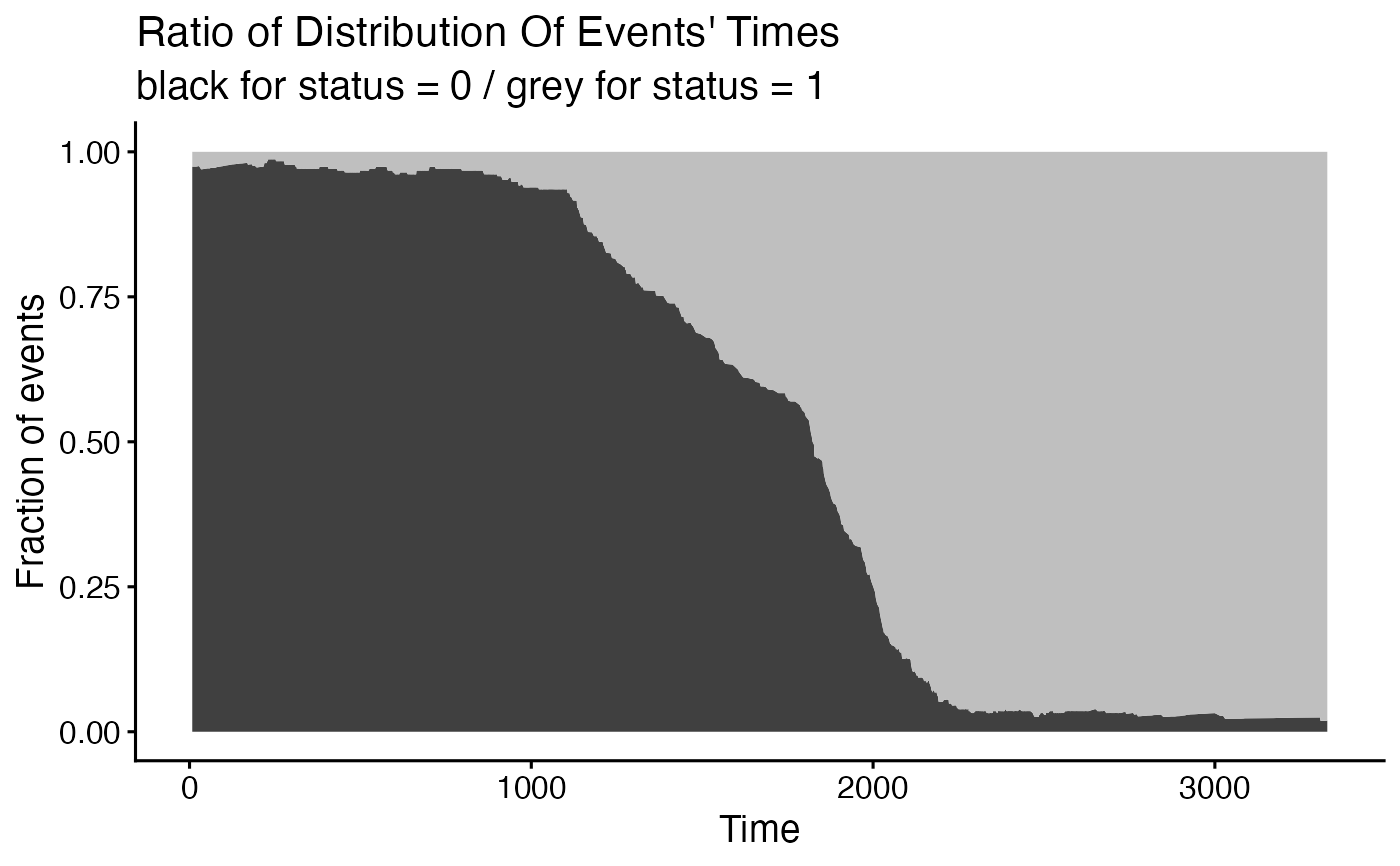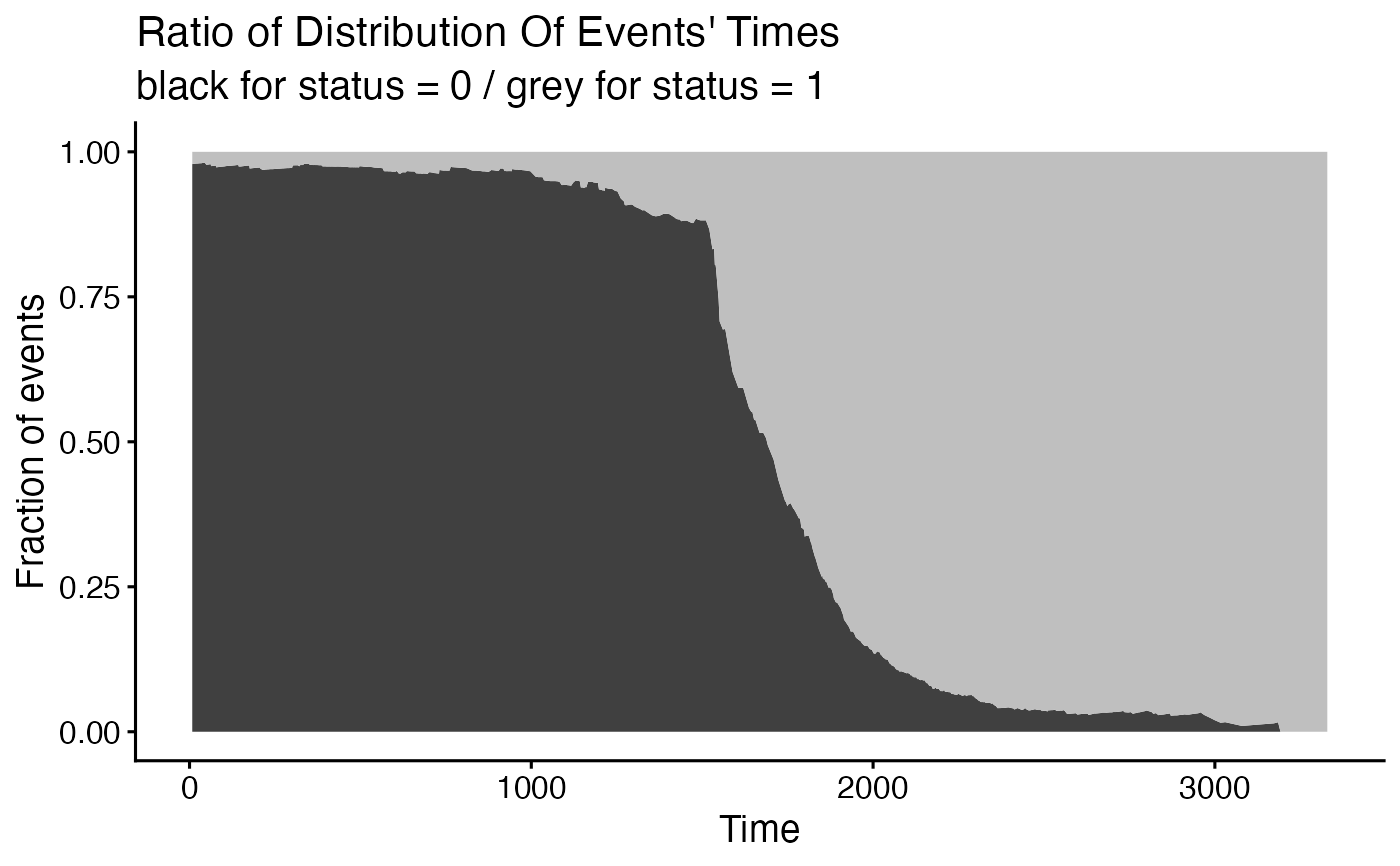Distribution of Events' Times

ggsurvevents(
surv = NULL,
fit = NULL,
data = NULL,
type = "fraction",
normalized = TRUE,
censored.on.top = TRUE,
ggtheme = theme_survminer(),
palette = c("grey75", "grey25"),
...
)

## Arguments

surv an object of Surv. If not suplied, the censoring variable is extracted from the model. an object of class survfit. a dataset for predictions. If not supplied then data will be extracted from fit object. one of c("cumulative", "radius", "fraction"). "cumulative" stands for cumulative number of events, "radius" stands for number of events within a given radius, if TRUE relative number of events is presented, is TRUE then censored events are on the top function, ggplot2 theme name. Allowed values include ggplot2 official themes: see theme. the color palette to be used for coloring of significant variables. other graphical parameters to be passed to the function ggpar.

## Value

return an object of class ggplot

## Examples

require("survival")
# from Surv
surv <- Surv(lung$time, lung$status)
ggsurvevents(surv)surv2 <- Surv(colon$time, colon$status)
ggsurvevents(surv2)ggsurvevents(surv2, normalized = TRUE)# from survfit
fit <- survfit(Surv(time, status) ~ sex, data = lung)
ggsurvevents(fit = fit, data = lung)#> Warning: The surv argument is not provided. The censored variable will be extracted from model fit.# from coxph
model <- coxph( Surv(time, status) ~ sex + rx + adhere, data = colon )
ggsurvevents(fit = model, data = colon)#> Warning: The surv argument is not provided. The censored variable will be extracted from model fit.ggsurvevents(surv2, normalized = TRUE, type = "radius")ggsurvevents(surv2, normalized = TRUE, type = "fraction")